Courses

# Chapter Notes - Structure of the Atom Class 9 Notes | EduRev

## Class 9 : Chapter Notes - Structure of the Atom Class 9 Notes | EduRev

The document Chapter Notes - Structure of the Atom Class 9 Notes | EduRev is a part of the Class 9 Course Science Class 9.
All you need of Class 9 at this link: Class 9

1. A major challenge before the scientists at the end of 19th century was to reveal the structure of the atom as well as to explain its important properties. The elucidation of structure of atoms is based on a series of experiments.
2. One of the first indications that atom is not indivisible comes from studying static electricity and the condition under which electricity is conducted by different substances.
3. There are charged particles in the atom, positively and negatively charged.

Activity: Perform an activity to prove that atom consists of charged particles.
Materials required:
Comb, pieces of paper, glass rod, silk cloth, inflated balloon.
(i) Comb dry hair.
(ii) Bring the comb near small pieces of paper.
(iii) Now rub the glass rod with silken cloth.
(iv) Bring the rod near an inflated balloon.
Observations: We observe that the pieces of paper are attracted towards the comb.
Similarly, the inflated balloon is attracted towards the glass rod.
Conclusion: These observations prove that comb and glass rod get electrically charged on rubbing with dry hair and silk cloth respectively. And they attract pieces of paper and inflated balloon respectively.
This proves that atom consists of charged particles.

4. J.J. Thomson in 1900 identified sub-atomic particle - electron which is negatively charged and is a constituent of atom.
5. E. Goldstein in 1886 discovered the existence of positively charged particles in the form of canal rays in a gas discharge. The positively charged particles (protons) had a charge equal in magnitude but opposite in sign to that of electron. The mass of positively charged particle is 2000 times that of the electron.
6. The mass of electron is negligible but its charge is taken as - 1. It is represented as e-. The mass of positively charged particle is taken as one unit and the charge assigned to it +1. It is represented as p+.
7. Thomson model of an atom: Thomson proposed a model of atom similar to that of Christmas pudding. The electrons, in a sphere of positive charge, were like currants (dry fruits) in a spherical Christmas pudding. We can also think of atom as watermelon; where positive charge in spread all over like the red edible part of the watermelon, while the electrons are studded in the positively charged sphere, like the seeds in the watermelon as shown in figure.
Thomson proposed that:
(i) An atom consists of a positively charged sphere and the electrons are embedded in it.
(ii) The negative and positive charges are equal in magnitude. So the atom as a whole is electrically neutral.
But Thomson model could not explain the results of various experiments.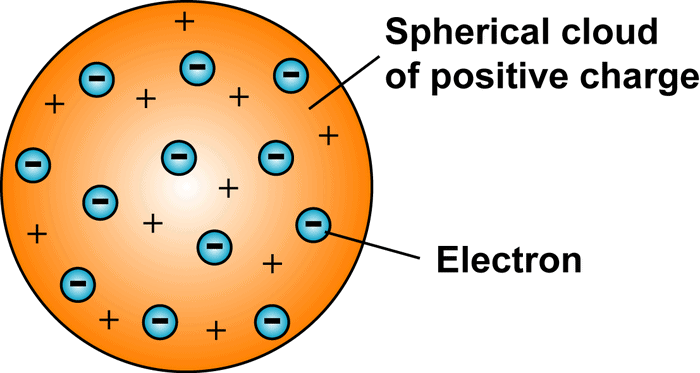Fig 4.1:Thomson's Model of an Atom

8. Rutherford’s model of Atom: Rutherford performed an experiment to arrive at the arrangement of particles in an atom. He bombarded a gold foil with fast moving a-particles. a-particles are helium nuclei with a mass of 4 u and a charge of +2. The following observations were made :
(i) Most of the fast moving a-particles passed straight through the gold foil (see Figure).
(ii) Some of the particles were deflected by the foil by small angles.
(iii) One out of 12000 particles appeared to rebound.
Rutherford made the following conclusions from the scattering experiment:
(i) Most of the space inside the atom is empty because most of the a-particles passed through the gold foil without getting deflected.
(ii) Very few particles were deflected from their path, indicating that the positive charge of the atom occupies very little space
(iii) A small fraction of a-particles were deflected by 180°, indicating that all the positive charge and the mass of the atom were concentrated in a very small volume within the atom.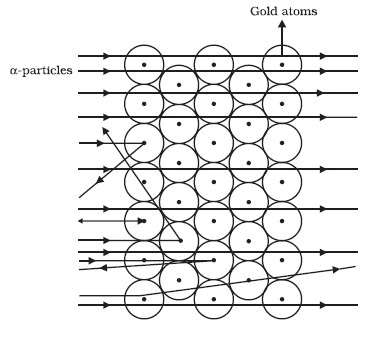Fig: 4.2 Scattering of α-particles by a Gold FoilOn the basis of his conclusions on the scattering experiment, Rutherford put forward his theory of structure of atom. Main points of the theory are:
(i) There is a positively charged centre in an atom called the nucleus. Nearly all the mass of an atom resides in the nucleus.
(ii) The electrons revolve around the nucleus in well-defined orbits.
(iii) The size of the nucleus is very small as compared to the size of the atom.
9. Drawbacks of Rutherford's Theory: Any charged particle which is moving in a circular path would lose energy and finally fall into the nucleus. This would lead to destruction of atom. But we know that this does not happen. Atom is quite stable. Rutherford model does not explain this anomaly.
10. Bohr’s model of an atom: To explain the objections against Rutherford model of structure of atom, Bohr put forward his theory of structure of atom. Main points of the theory are:
(i) Only certain special orbits, known as discrete orbits of electrons are allowed inside the atom.
(ii) While revolving in discrete orbits, the electrons do not radiate energy.
These orbits or shells are called energy levels, which are shown in Fig. 4.3.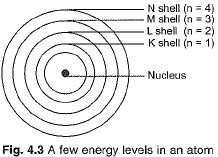11. Discovery of neutrons: In 1932, J. Chadwick discovered particles in atom, which had no charge and a mass equal to that of a proton. It was given the name neutron. It is represented by ‘n’ Mass of an atom is given by the sum of the masses of protons and neutrons present in the nucleus.
12. Distribution of electrons in different orbits (shells): Distribution of electrons in the orbits of an atom is governed by Bohr-Bury rules. These rules are:
(i) The maximum number of electrons present in a shell is given by 2n2 where n is the orbit number like 1, 2, 3,.......... Thus, the maximum number of electrons in different orbits can be calculated as under:
In first orbit or K-shell (n-1)=2 x n2 =2 x 12 =2
In second orbit or L-shell (n-2)=2 x n2 =2 x 22 =8
In third orbit or M-shell (n-3)=2 x n2 =2 x 32 =18
and so on.
(ii) But, the maximum number of electrons that can be accommodated in the outermost orbit is 8.
(iii) Electrons are not accommodated in a given shell, unless the inner shells are filled.
13. Structure of first eighteen elements from hydrogen to argon: Atomic structures of first eighteen elements showing the number of electron s in different orbits or shells, is given in Fig. 4.4.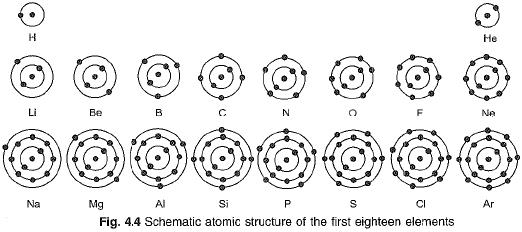14. Valency: According the Bohr-Bury scheme of filling of orbitals, the outermost orbit cannot have more than 8 electrons. It was also observed the elements having completely filled outermost orbit show little chemical activity. Valency of such elements is zero. It has been felt that substances react in order to attain a fully filled outermost shell. An outermost shell which has eight electrons is said to possess an octet. Thus atoms react in order to achieve octet. This can be done by gaining, losing or sharing electrons. For example, elements like hydrogen, sodium and potassium can complete their octet (8 electrons in the outermost shell) by losing one electron. So these elements are univalent. Similarly magnesium and aluminium have valancy two and three respectively because they have twb and three electrons respectively in their outermost shell.
We can say that for elements of groups 1, 2, 13, the valency is the number of electrons in the valence shell. For group 14, where the number of electrons in the outermost shell is 4, the valency is 4. However, these elements neither have the tendency to lose electrons nor to gain electrons. For group 15, 16 and 17 elements, the valency is eight minus the number of electrons in the valence shell.
15. It is not always possible to lose electrons. For example, fluorine atom has 7 electrons in the outermost shell. To complete the octet, it may have to lose 7 electrons. But, it is easier for it to gain 1 electron. Thus its valency is 1.
Valency of first eighteen elements is given in Table 4.1 below: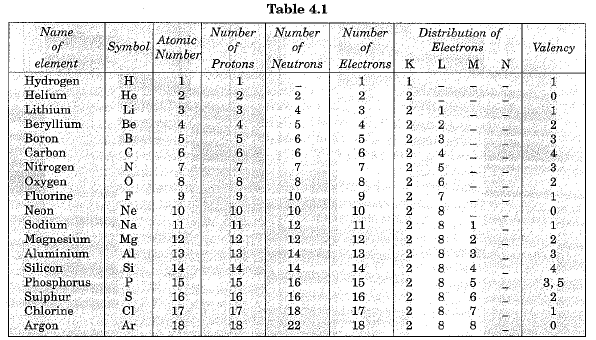16. Atomic Number: Atomic number of an element is equal to the number of protons present in the nucleus of an atom of the element. It is denoted by Z. For hydrogen Z = 1 because there is only one proton in the nucleus of the atom. Similarly for carbon, Z = 6.
17. Mass Number: Mass number of an element is the sum of the total number of protons and neutrons present in the nucleus of the atom. For example, mass number of carbon is 12 because it has 6 protons and 6 neutrons in the nucleus. Protons and neutrons together are called nucleons. In the notation for an atom, the atomic number, mass number and the symbol of the element are to be written as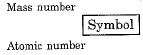For example, nitrogen as written as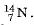18. Isotopes: Isotopes are defined as the atoms of the same element having the same atomic number but different mass numbers.
Hydrogen has three isotopes namely protium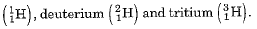Protium, deuterium and tritium are also represented by H, D and T respectively. Chlorine occurs in nature in two isotopic forms with masses 35 u and 37 u in the ratio of 3 : 1. Mass of an atom of any natural element is taken as the average mass of all the naturally occurring atoms of that element. The average atomic mass of chlorine atom can be calculated as under :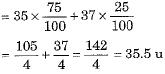19. Applications of isotopes: Isotopes have the following applications:
(i) An isotope of uranium is used as a fuel in nuclear reactors.
(ii) An isotope of cobalt is used in the treatment of cancer.
(iii) An isotope of iodine is used in the treatment of goiter.
20. Isobars: The element calcium (Ca) posseses atomic number 20 and the element argon (Ar) posseses atomic number 18. The number of electrons in both these elements is different. But the mass number of both the elements is 40. Thus, the total number of nucleons (protons and neutrons) is the same in the two atoms. Such atoms of different elements with different atomic numbers but having the same mass number are called isobars.

Offer running on EduRev: Apply code STAYHOME200 to get INR 200 off on our premium plan EduRev Infinity!

## Science Class 9

90 videos|226 docs|135 tests

,

,

,

,

,

,

,

,

,

,

,

,

,

,

,

,

,

,

,

,

,

;